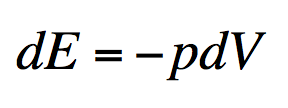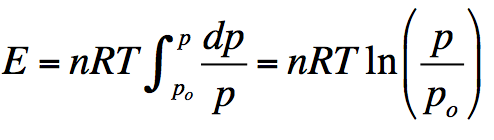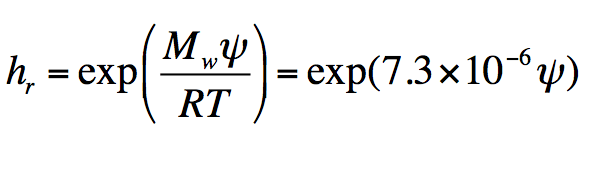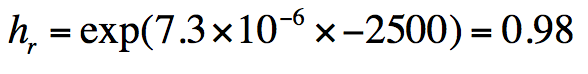# Estimating Relative Humidity in Soil: How to Stop Doing it Wrong

Estimating the relative humidity in soil?  Most people do it wrong…every time.  Dr. Gaylon S. Campbell shares a lesson on how to correctly estimate soil relative humidity from his new book, Soil Physics with Python, which he recently coauthored with Dr. Marco Bittelli.

A number of years ago a former student told me of a meeting he had with some engineers establishing a low-level radioactive waste repository in a desert area. The waste was to be buried, and at least some of it was in steel containers which would corrode if the humidity was too high. The engineers assumed the humidity in the soil would be pretty low because it was a desert, but they didn’t know how low. So, what is the relative humidity in soil? That sounds like it would be a hard thing to find out without measuring it, but it isn’t. Let’s apply a little physics to see what we can find.

The energy required to create an infinitesimal volume of water vapor can be found using the first law of thermodynamics. For an adiabatic systemwhere dE is the energy required, p is the pressure, and dV is the volume change.

The Boyle-Charles law, which gives the pressure-volume relationship for a perfect gas, iswhere n is the number of moles of gas, R is the universal gas constant, and T is the kelvin temperature. Rearranging terms and taking the derivative of both sides givesThis equation can be substituted for dV in the first equation, givingThe total energy required to go from a reference vapor pressure, po (the vapor pressure of pure water) to the vapor pressure of the water in the soil, p isWe can divide both sides by the mass of water. The left side then becomes the energy per unit mass of water in the soil, which we call the water potential. On the right side, the number of moles per unit mass is the reciprocal of the molecular mass of water, and the ratio of the vapor to the saturation vapor pressure is the relative humidity hr so the final equation isWe can rearrange this and take the exponential of both sides, givingIn the second version of the equation the molecular mass of water, the gas constant and the temperature (298K) have been substituted.

We can use this equation to find the range of humidities we would expect in soil. When soil is very wet, the water potential is near 0, so the humidity is exp(0) = 1. At the dry end, the soil is dried mainly by plant water uptake. Even desert soils support some vegetation. The soil near the surface will be dried by evaporation, but a few decimeters below the surface the lowest water potentials are those to which plants can dry them. The nominal permanent wilting point (lower limit of plant available water) is -1500 J/kg. Desert vegetation can extract water to lower potentials. If we say their lower limit is -2500 J/kg, then the humidity isso the relative humidity in the soil is around 98%. Sagebrush can go lower than -2500 J/kg. We measured -7000 J/kg under it at the end of the growing season. Even that, though, is around 95% humidity.

The conclusion is that the humidity in the soil is always near saturation, except in a shallow evaporation layer near the surface. I don’t remember what the engineers were expecting. I think anything above 60 or 70% was going to be a disaster for corroding the steel containers. I don’t know whether they believed the calculations or just went on thinking that desert soil is dry.

1.